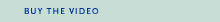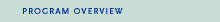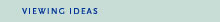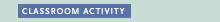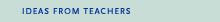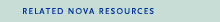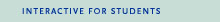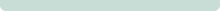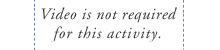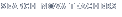Back to Teachers HomeThree Men and a BalloonClassroom ActivityObjective
To use visual perspective to estimate distance.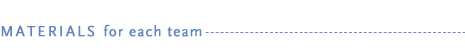• copy of "At Arm's Length" student handout (PDF or HTML)
• String—at least 10 meters (30 feet)
• Ruler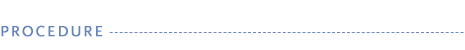1. Although the crew members in this program rely on sophisticated equipment to calculate their path, there are simpler ways of determining altitude, distance, and size of objects which some amateur balloonists use.

2. To give students experience in using their own visual perspective to estimate measurements, try this activity.

3. Group students into teams and distribute a copy of the "At Arm's Length" student handout to each student and a set of materials to each pair.

4. Have students work in pairs on Part I, beginning with an object that is four to five inches wide so they can see the patterns in their chart and work with the distance formula in Part II. Students who understand the concept can go on to calculate estimated distances when 2 thumbs = 1 target, 1/2 thumb = 1 target, and 1/3 thumb = 1 target, and try to come up with a general formula to satisfy all cases.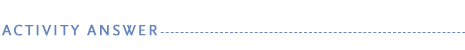The key to this activity is to find the number of thumbs at arm's length that equal the width of a target in the distance. The distance between the student and the target is inversely proportional to the number of thumb widths: the fewer thumbs that appear to equal the width of the target, the farther away the student is. The more thumbs that seem to fit into the target, the closer the student is.

If student measurements seem dramatically different than expected, check to make sure that they used consistent units of measure for the entire calculation and that they are consistent about comparing the same width and portion of their thumbs and the same width and portion of their targets.

General equations to satisfy all cases are as follows:

If thumb and target appear to be the same width,

d = N x A.

If thumb appears to be wider than target,

d = N x A x number of times thumb is wider.

If target appears to be wider than thumb,

d = (N x A) / number of times object is wider.

Students can also estimate distances by using other objects besides their thumbs at arm's length, such as a coin, a pencil, or a book. All they need to do is calculate new values for B, C, and N.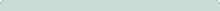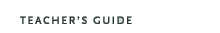Three Men and a Balloon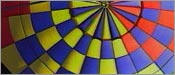Original broadcast:October 15, 1996